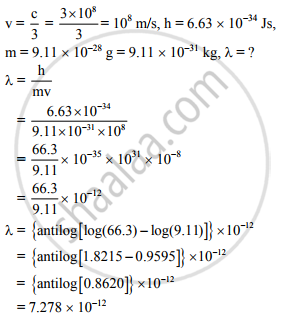HSC Science (Computer Science) 12th Board ExamMaharashtra State Board
Share

Calculate the De Broglie Wavelength of an Electron Moving with - of the Speed of Light in Vacuum (Negelct Relativistic Effect) - HSC Science (Computer Science) 12th Board Exam - Physics

Question

Calculate the de Broglie wavelength of an electron moving with - of the speed of light in vacuum (Negelct relativistic effect)

(Planck's constant: h = 6.63 x 10-34 Js, Mass of electron : m = 9.11 x 10-28 g)

Solution∴ The de Broglie wavelength of electron is 7.278 × 10−12 m

Is there an error in this question or solution?

APPEARS IN

2013-2014 (October) (with solutions)
Question 6.8 | 2.00 marks

Video TutorialsVIEW ALL 

Solution Calculate the De Broglie Wavelength of an Electron Moving with - of the Speed of Light in Vacuum (Negelct Relativistic Effect) Concept: de-Broglie Relation.
S